# Texas Go Math Grade 3 Lesson 1.4 Answer Key Numbers Through Ten Thousands on a Number Line

Refer to our Texas Go Math Grade 3 Answer Key Pdf to score good marks in the exams. Test yourself by practicing the problems from Texas Go Math Grade 3 Lesson 1.4 Answer Key Numbers Through Ten Thousands on a Number Line.

## Texas Go Math Grade 3 Lesson 1.4 Answer Key Numbers Through Ten Thousands on a Number Line

Essential Question
How can you represent numbers through ten thousands on a number line to round whole numbers?

Unlock the Problem

When you round a number, you find a number that tells you about how much or about how many.

Maya’s collie weighs 68 pounds. What is its weight rounded to the nearest ten pounds?

Use a number line to round.
A. Round 68 to the nearest ten.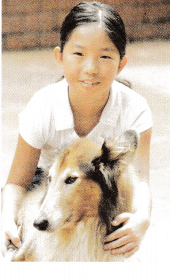Find which tens the number is between.
68 is between ___ and ___.
68 is closer to ___ than it is to ___.
So, 68 rounded to the nearest ten is

68 is between 60 and 70.
68 is closer to 70 than it is to 60.
So, 68 rounded to the nearest ten is 70

B. Round 327 to the nearest hundred.Find which hundreds the number is between.

327 is between ____ and ____.
327 is closer to ____ than it is to ____.
So, 327 rounded to the nearest hundred is _____.

327 is between 300  and 400.
327 is closer to 300 than it is to 400.
So, 327 rounded to the nearest hundred is 300

Math talk
Mathematical Processes
Explain how locating 327 on a number line helps you understand the size of the number.

C. Round 7,452 to the nearest thousand.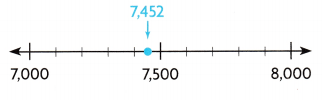7,452 is between ___ and ____.
7,452 is closer to ____ than it is to ___.
So, 7,452 rounded to the nearest thousand is ____.

7,452 is between 7000 and 8000.
7,452 is closer to 7000 than it is to 8000.
So, 7,452 rounded to the nearest thousand is 7000.

D. Round 24,615 to the nearest ten thousand.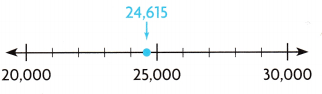24,615 is between ___ and ___.
24,6 1 5 is closer to ____ than it is to ____.
So, 24,615 rounded to the nearest ten thousand is ___.

24,615 is between 20,000 and 30,000.
24,6 1 5 is closer to20,000 than it is to 30,000.
So, 24,615 rounded to the nearest ten thousand is 30,000

Share and Show

Use the number line for 1-2. Locate 8,714 on the number line. Round 8,714 to the nearest thousand.

Math Talk
Mathematical Processes

What is the greatest number that rounds to 9,000 when rounding to the nearest thousand? What is the least number?

Question 1.
8,714 is between ___ and ____

8,714 is between 8000 and 9,000.
8,714 is closer to 9,000 than it is to 8000.
So, 8,714 rounded to the nearest thousand is 9000.

Question 2.
8,714 is closer to ___ than it is to _____

8,714 is closer to 9,000 than it is to 8000.

Round to the nearest ten.

Question 3.
19 ____

19 is between 10 and 20.
19 is closer to 20 than it is to 10.
So, 19 rounded to the nearest ten is 20

Question 4.
51 ______

51 is between 50 and 60.
51 is closer to 50  than it is to 60.
So, 51 rounded to the nearest ten is 50

Round to the nearest hundred.

Question 5.
457

457 is between 400 and 500.
457 is closer to 500 than it is to 400.
So, 457 rounded to the nearest hundred is 500.

Question 6.
202

202 is between 200 and 300.
202 is closer to 200 than it is to 300.
So, 202 rounded to the nearest hundred is 200.

Round to the nearest ten thousand.

Question 7.
33,572

33,572 is between 30,000 and 40,000.
33,572 is closer to 40,000 than it is to 30,000.
So, 33,572 rounded to the nearest ten thousand is 40,00.

Question 8.
62,276

62,276 is between 60,000 and 70,000.
62,276 is closer to 60,000 than it is to 70,000.
So, 62,276 rounded to the nearest ten thousand is 60,000.

Question 9.
98,471

98,471 is between 90,000 and 1,00,000.
98,471 is closer to 90,000 than it is to 1,00,000.
So, 98,471 rounded to the nearest ten thousand is 1,00,000.

Question 10.
Multi-Step Sandy works at a zoo. She records the number of visitors to the giraffe exhibit each day. The table shows her records for one week. On which two days did about 800 visitors come to the giraffe exhibit?a. What do you need to find?

We need to find On which two days did about 800 visitors come to the giraffe exhibit

b. What information are you given?

The given information contains the number of visitors to the giraffe exhibit each day.

c. What plan or strategy you will use to solve the problem?

We can compare hundreds place and tens place to find the number which are about 800 visits for two days.

Complete the sentences, ___ and __ would be 800 when rounded to the nearest hundred.
So, the number of visitors on __ and ___ is about 800 each day.

763 and 839 would be 800 when rounded to the nearest hundred.
So, the number of visitors on Monday and Thursday is about 800 each day.

Question 11.
H.O.T. How can you use a number line to help you understand the size of the number 13,700? Explain.Question 12.
H.O.T. Multi-Step Round 46952 to the nearest ten, to the nearest hundred, to the nearest thousand, and to the nearest ten thousand.

46952

Nearest ten = 50

Nearest hundred = 1000

Nearest thousand = 7000

Nearest ten thousand = 50,000Fill in the bubble for the correct answer choice.

Question 13.
Use Tools A contractor covers an office building floor with 52,340 small tiles. Which point on the number line shows the number of tiles?(A) point A
(B) point B
(C) point C
(D) point D

For the number 52,340 nearest ten thousand 50,000

50,000 lies on the point C

Therefore, point C on the number line shows the number of tiles.

Question 14.
Between which two thousands is 7,248 on the number line?(A) 8,000 and 9,000
(B) 7,000 and 8,000
(C) 6,000 and 7,000
(D) 9,000 and 10,000

On comparing thousand and hundreds place 7,248 is near to 7,000 and 8,000

Therefore, Between 7,000 and 8,000, thousands is 7,248 on the number line.

Question 15.
Multi-Step One ranch is home to 463 cattle. Another ranch is home to 517 cattle. Which number on the number line is closest to the number of cattle at each ranch?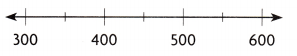(A) 400
(B) 600
(C) 500
(D) 300

Number of cattle in one ranch = 463

Number of cattle in another ranch = 517

On comparing Hundreds place and tens place,

463, 517 numbers are near to 500

Therefore, 500 is the the number on the number line is closest to the number of cattle at each ranch.

Texas test prep

Question 16.
What is 438 rounded to the nearest hundred?
(A) 440
(B) 4,000
(C) 400
(D) 500

On comparing hundreds place and tens place,

438 is near to 400

Therefore, 438 nearest hundred is 400.

### Texas Go Math Grade 3 Lesson 1.4 Homework and Practice Answer Key

Round to the nearest thousand.

Question 1.
3,489

3489 is between 3000 and 4,000.
3,489 is closer to 3,000 than it is to 4000.

3,489 nearest thousand is 3,000

Question 2.
5,890

5,890 is between 5000 and 6,000.
5,890 is closer to 6,000 than it is to 5000.

5890 nearest thousand is 6,000

Question 3.
1,045

1,045 is between 1000 and 2,000.
1,045 is closer to 1,000 than it is to 2000.

1,045 nearest thousand is 1,000

Question 4.
6,589

6,589 is between 6000 and 7000.
6,589 is closer to 7000 than it is to 6000.

6,589 nearest thousand is 7000

Round to the nearest ten thousand.

Question 5.
15,677

15,677 is between 10,000 and 20,000.
15,677 is closer to 20,000 than it is to 10,000.

15,677 nearest ten thousand is 20,000

Question 6.
30,944

30,944 is between 30,000 and 40,000.
30,944 is closer to 30,000 than it is to 40,000.

30,944 nearest ten thousand 30,000

Question 7.
87,142

87,142 is between 80,000 and 90,000.
87,142 is closer to 90,000 than it is to 80,000.

87,142 nearest ten thousand is 90,000

Question 8.
62,763

62,763 is between 60,000 and70,000
62,763 is closer to  60,000 than it is to 70,000 .

62,763 nearest ten thousand 60,000

Question 9.
Round 47,125 to the nearest ten, to the nearest hundred, to the nearest thousand, and to the nearest ten
thousand.

47,125

Nearest ten = 30

Nearest hundred = 100

Nearest thousand = 7000

Nearest ten thousand = 50,000

Question 10.
Round 63,849 to the nearest ten, to the nearest hundred, to the nearest thousand, and to the nearest ten thousand.

63,849

Nearest ten = 50

Nearest hundred = 800

Nearest thousand = 4000

Nearest ten thousand = 60,000

Problem solving.

Question 11.
Gina has 237 baseball cards. Ross has 418 baseball cards. Jaquel has 387 baseball cards. Which two children have about 400 baseball cards?

Given,

The number of baseball cards Gina have = 237

The number of cards Ross have = 418

The number of cards Jaquel have = 387

On comparing hundreds place and tens place we can find out Which two children have about 400 baseball cards

418, 387 are near to 400

Which means, Ross and Jaquel have about 400 baseball cards.

Question 12.
A bookstore sells 617 books on Monday, 498 books on Tuesday, and 563 hooks on Wednesday. On which two days did the bookstore sell about 600 books?

Given, The number of books bookseller sells on Monday = 617

The number of books sold on Tuesday = 498

The number of books sold on Wednesday = 563

On comparing Hundreds place we can conclude.

According the given condition, 617, 563 are near to 600

Which means, On Monday And Wednesday the bookstore sell about 600 books.

Lesson Check

Texas Test prep

Question 13.
A ranch has 3,475 cattle. Between which two thousand is this number?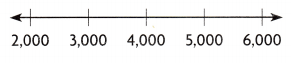(A) 2,000 and 3,000
(B) 4,000 and 5,000
(C) 3,000 and 4,000
(D) 5,000 and 6,000

On comparing thousands place and hundreds place,

3,475 is between 3000 and 4000.

Question 14.
Meg has saved 645 pennies. Between which two hundred is 645?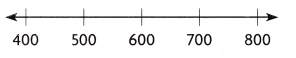(A) 600and 700
(B) 700 and 800
(C) 400 and 500
(D) 500 and 600

The number of pennies Meg have = 645

645 is between 600 and 700

On comparing Hundreds place we can conclude.

Therefore, 645 is between 600 and 700.

Question 15.
An orchard produces 34,198 oranges. Which point on the number line shows this number?(A) point A
(B) point B
(C) point C
(D) point D

Point C

On comparing Ten thousand place and thousands place, 34,198 is near to 30,000

30,000 lies on point C on the number line.

Therefore, Point C on the number line shows this number.

Question 16.
An airplane travels 6,779 miles. Which point on the number line shows this number?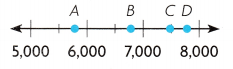(A) point A
(B) point B
(C) point C
(D) point D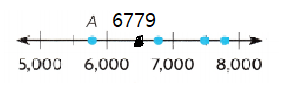On comparing thousands place and  Hundreds place

6,779 is near to 7,000 Therefore, the number lies on point B.

Question 17.
Multi-Step At the early movie show, there are 316 people. At the late show, there are 278 people. Which number is closest to the number of people at each show?
(A) 400
(B) 200
(C) 1oo
(D) 300

Given, The number of people there for early show = 316

The number of people there for late show = 278

On comparing hundreds place, the nearest hundred is 300

Therefore, 300 is closest to the number of people at each show

Question 18.
Multi-Step In May a store sells 4,178 cartons of milk. In June the store sells 3,816 cartons of milk. Which number is closest to the number of cartons sold each month?
(A) 5,000
(B) 2,000
(C) 3,000
(D) 4,000

Explanation:

Given,

The amount of milk sold in the month of May = 4,178 cartons

The amount of milk sold in the month of June = 3,816 cartons

The thousand which is near to both values =

4,178 is nearest to 4,000

3,816 is also near to 4000

Therefore, 4000 is closest to the number of cartons sold each month.

Scroll to Top